# 2.5D(伪3D)站点可视化第一弹

2019/04/10 10:10

#楔子 最近要做一个基站站点的可视化呈现项目。 我们首先尝试的是三维的可视化技术来程序，但是客户反馈的情况是他们的客户端电脑比较差，性能效率都会不好，甚至有的还是云主机。 因此我们先做了一个性能比较极致的3Ddemo，如下图所示：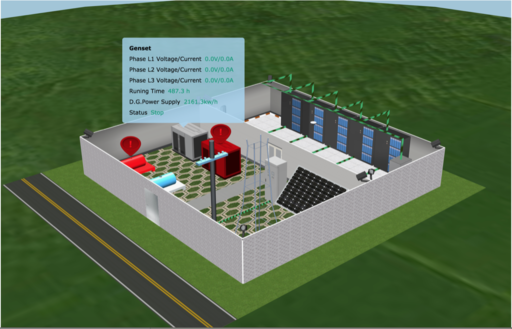# 2.5D的思想火花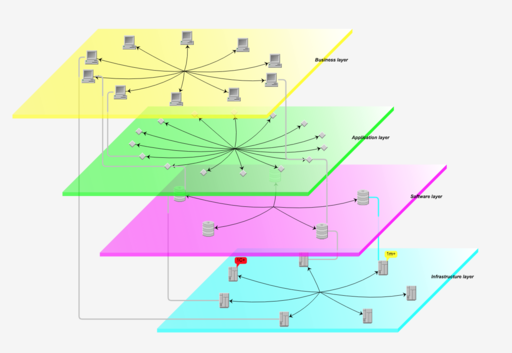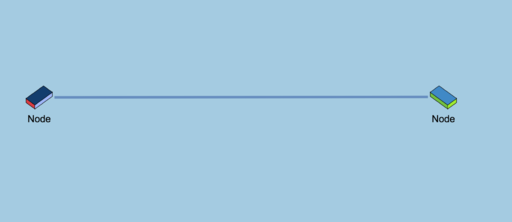# 2.5D技术概述

• 三维空间的定义
• 模型的定义（使用三维空间坐标定义模型）
• 投影算法 把三维空间的坐标点通过投影算法，转换为二维空间的坐标。

# 模型定义

  setSize3: function(w, h, d) {
var oldValue = {
w: this._width3,
h: this._height3,
d: this._depth3,
};
this._width3 = w;
this._height3 = h;
this._depth3 = d;
this.firePropertyChange('size3', oldValue, { w: w, h: h, d: d });
},


 setPosition: function(x, y, z) {
var oldValue = this.getPosition();
this._position = {
x: x,
y: y,
z: z,
};
this.firePropertyChange('position', oldValue, this._position);
},


# 投影算法。

 /**
* 计算变换矩阵，变换矩阵由镜头参数决定
*/
calMVMatrix: function() {
var angle = this.getAngle3(),
vAngle = this.getVAngle3(),
viewMatrix = mat4.create(),
projectMatrix = mat4.create(),
mvMatrix = mat4.create(),
winWidth = 1,
winHeight = 1;
mat4.lookAt(
viewMatrix,
[
radius * Math.cos(vAngle) * Math.sin(angle),
radius * Math.cos(vAngle) * Math.cos(angle),
],
[0, 0, 0],
[0, 1, 0]
);
mat4.ortho(
projectMatrix,
-winWidth / 2,
winWidth / 2,
-winHeight / 2,
winHeight / 2,
0.1,
1000
);
mat4.multiply(mvMatrix, projectMatrix, viewMatrix);
this.mvMatrix = mvMatrix;
},



/**
* 布局，前面四个点 p1 - p4, 后面 四个点p 5 - p8
*
*          p8   p7
*
*          p5   p6
*
*     p4   p3
*
*     p1   p2
*
*/
var points1 = [
{
x: -w3 / 2 + pos.x,
y: -h3 / 2 + pos.y,
z: d3 / 2 + pos.z,
}, // p1
{
x: w3 / 2 + pos.x,
y: -h3 / 2 + pos.y,
z: d3 / 2 + pos.z,
}, // p2
{
x: w3 / 2 + pos.x,
y: h3 / 2 + pos.y,
z: d3 / 2 + pos.z,
}, // p3
{
x: -w3 / 2 + pos.x,
y: h3 / 2 + pos.y,
z: d3 / 2 + pos.z,
}, // p4
{
x: -w3 / 2 + pos.x,
y: -h3 / 2 + pos.y,
z: -d3 / 2 + pos.z,
}, // p5
{
x: w3 / 2 + pos.x,
y: -h3 / 2 + pos.y,
z: -d3 / 2 + pos.z,
}, // p6
{
x: w3 / 2 + pos.x,
y: h3 / 2 + pos.y,
z: -d3 / 2 + pos.z,
}, // p7
{
x: -w3 / 2 + pos.x,
y: h3 / 2 + pos.y,
z: -d3 / 2 + pos.z,
}, // p8
];

var points = (this._points = []);

points1.forEach(function(point) {
var newPoint = self.getPositionByRotate(
point,
pos,
rotationX,
rotationY,
rotationZ
);
points.push({
x: newPoint,
y: newPoint,
z: newPoint,
});
});

var ps = (this._projectPoints = points.map(function(point) {
return self.getProjectionPoint(point);
}));



## 绘制边框效果

drawPoints: function (ctx, points, close, dash, fill, borderColor, image) { if (!points || points.length == 0) { return; } ctx.beginPath(); ctx.strokeStyle = "black"; if (borderColor) { ctx.strokeStyle = borderColor; } ctx.lineWidth = 1; ctx.fillStyle = 'rgb(102,204,255)'; if (dash) { ctx.setLineDash([4, 4]); ctx.strokeStyle = 'rgba(0,0,0,0.5)'; } else { ctx.setLineDash([1, 0]); } ctx.moveTo(points.x, points.y);

    for (var i = 1; i < points.length; i++) {
var p = points[i];
ctx.lineTo(p.x, p.y);
}

if (close) {
ctx.lineTo(points.x, points.y);
}

ctx.closePath();
ctx.stroke();


} 最终的绘制效果如下图所示：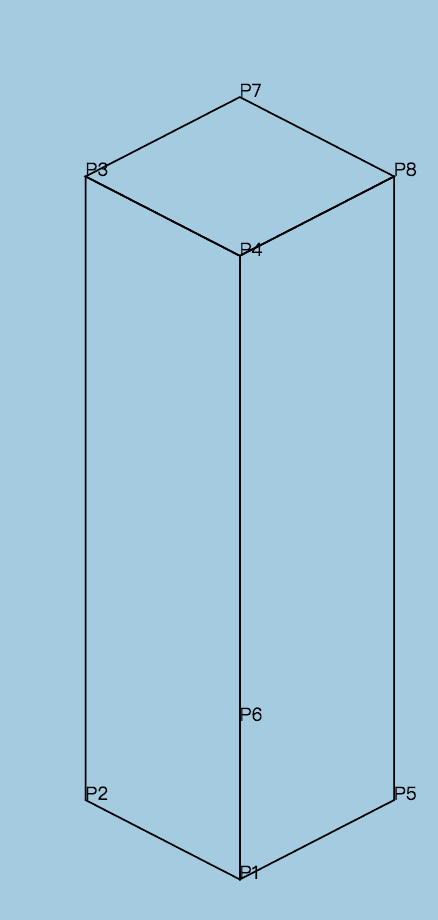## 绘制颜色填充效果

  if (fill) {
ctx.fill();
// drawImageInPoints(ctx, image, points);
}


## 绘制图片

  /**
* 根据points中的8个点，找出包裹8个点的最小rect
*
* @param {Array} points - 8个点的2d坐标
* @returns {Object} - rect
*/
getRect: function(points) {
var minX, minY, maxX, maxY;
points.forEach(function(point) {
if (minX == null) {
minX = maxX = point.x;
minY = maxY = point.y;
} else {
minX = Math.min(minX, point.x);
maxX = Math.max(maxX, point.x);
minY = Math.min(minY, point.y);
maxY = Math.max(maxY, point.y);
}
});
return {
x: minX,
y: minY,
width: maxX - minX,
height: this.getElement().getClient('reflect')
? (maxY - minY) * 2
: maxY - minY,
};
},


 ctx.drawImage(
image,
0,
image.height - 20,
image.width,
20,
rect.x,
rect.y + rect.height - 20,
rect.width,
20
);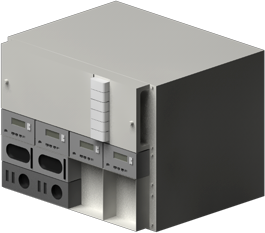## 搭建地面、墙面

 var node1 = new twaver.Node2_5({
styles: {
'body.type': 'vector',
},
name: 'TWaver',
centerLocation: {
x: 300,
y: 200
},
width: 800 / 1,
height: 360 /.775,
});
node1.setImage(null);
node1.setPosition(00,0,100);
node1.setWidth3(1000);
node1.setHeight3(10);
node1.setDepth3(1200);
// node1.setStyle('top.image','image0');  // ToDo 定义样式规则
// node1.setStyle('top.image.rule','pattern');

var node1 = new twaver.Node2_5({
styles: {
'body.type': 'vector',
},
name: 'TWaver',

});
node1.setImage(null);
node1.setPosition(-250,155,-500);
node1.setWidth3(500);
node1.setHeight3(300);
node1.setDepth3(1);

var node1 = new twaver.Node2_5({
styles: {
'body.type': 'vector',
},
name: 'TWaver',

});
node1.setImage(null);
node1.setPosition(250,105,-500);
node1.setWidth3(500);
node1.setHeight3(200);
node1.setDepth3(1);
node1.setStyle('front.image','weilan');  // ToDo 定义样式规则### 作者的其它热门文章

0
0 收藏

0 评论
0 收藏
0